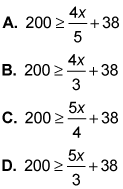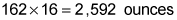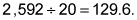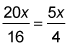##### SAT: 1,001 Practice Questions For DummiesA weight capacity question will probably appear on the SAT Math exam in the form of a word problem. The following practice questions give you the weight capacity of a table, and ask you to calculate the maximum number of bottles of soda the table can hold, and then build an equation that supports your answer.

## Practice questions

Questions 1 and 2 are based on the following information.

The weight capacity of a certain folding picnic table is 200 pounds. A cooler loaded with ice weighs 38 pounds.

1. If nothing else is on the table, what is the maximum number of unopened 20-ounce bottles of soda that can be placed on the table, along with the cooler, for the table to safely hold everything? Assume each bottle of soda weighs exactly 20 ounces. (16 ounces = 1 pound) A. 129 B. 130 C. 131 D. 132
2. Which of the following equations captures the scenario, where x represents the number of bottles of soda?1. The correct answer is Choice (A). If the cooler with ice weighs 38 pounds, the remaining table capacity is 162 pounds. The sodas are weighed in ounces, so the table hasremaining to hold sodas. Divide 2,592 by 20, the weight of each soda, for the number of bottles:The question asks for the number of unopened bottles, so drop the 0.6 for an answer of 129. Rounding up to 130 would exceed the capacity of the table.

Soda is usually measured in fluid ounces, a unit of volume, and a fluid ounce doesn't necessarily weigh 1 ounce. A 20–fluid ounce bottle of soda actually weighs a little over 21 ounces, so the real-world answer is a few bottles less. On the other hand, the table's weight capacity isn't exact.

2. The correct answer is Choice (C). Each soda weighs 20 ounces, so multiply x by 20 for the total number of ounces. Then divide this by 16 to convert it to pounds, and reduce the fraction:Add 38 to account for the weight of the cooler.